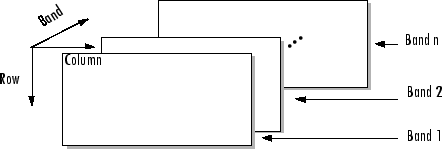Read band-interleaved data from binary file

## Syntax

```X = multibandread(filename,size,precision,offset,interleave,byteorder) X = multibandread(...,subset1,subset2,subset3) ```

## Description

`X = multibandread(filename,size,precision,offset,interleave,byteorder)` reads band-sequential (BSQ), band-interleaved-by-line (BIL), or band-interleaved-by-pixel (BIP) data from the binary file `filename`. The `filename` input is specified as a character vector or string scalar. This function defines band as the third dimension in a 3-D array, as shown in this figure.You can use the parameters of `multibandread` to specify many aspects of the read operation, such as which bands to read. See Parameters for more information.

`X` is a 2-D array if only one band is read; otherwise it is 3-D. `X` is returned as an array of data type `double` by default. Use the `precision` parameter to map the data to a different data type.

`X = multibandread(...,subset1,subset2,subset3) ` reads a subset of the data in the file. You can use up to three subsetting parameters to specify the data subset along row, column, and band dimensions. See Subsetting Parameters for more information.

Note

In addition to BSQ, BIL, and BIP files, multiband imagery may be stored using the TIFF file format. In that case, use the `imread` function to import the data.

## Parameters

This table describes the arguments accepted by `multibandread`.

Argument

Description

`filename`

Character vector or string scalar containing the name of the file to be read.

`size`

Three-element vector of integers consisting of `[height, width, N]`, where

• `height` is the total number of rows

• `width` is the total number of elements in each row

• `N` is the total number of bands.

This will be the dimensions of the data if it is read in its entirety.

`precision`

Character vector or string scalar specifying the format of the data to be read, such as `'uint8'`, `'double'`, `'integer*4'`, or any of the other precisions supported by the `fread` function.

Note: You can also use the `precision` parameter to specify the format of the output data. For example, to read `uint8` data and output a `uint8` array, specify a precision of `'uint8=>uint8'` (or `'*uint8'`). To read `uint8` data and output it in the MATLAB® software in single precision, specify `'uint8=>single'`. See `fread` for more information.

`offset`

Scalar specifying the zero-based location of the first data element in the file. This value represents the number of bytes from the beginning of the file to where the data begins.

`interleave`

Format in which the data is stored, specified as one of these values:

• `'bsq`' — Band-Sequential

• `'bil'`— Band-Interleaved-by-Line

• `'bip'`— Band-Interleaved-by-Pixel

For more information about these interleave methods, see the `multibandwrite` reference page.

`byteorder`

Character vector or string scalar specifying the byte ordering (machine format) in which the data is stored, such as

• `'ieee-le'` — Little-endian

• `'ieee-be'` — Big-endian

See `fopen` for a complete list of supported formats.

## Subsetting Parameters

You can specify up to three subsetting parameters. Each subsetting parameter is a three-element cell array, `{dim,method,index}`, where

Parameter

Description

`dim`

The dimension to subset along. Specified as any of these values:

• `'Column'`

• `'Row'`

• `'Band'`

`method`

The subsetting method. Specified as either of these values:

• `'Direct'`

• `'Range'`

If you leave out this element of the subset cell array, `multibandread` uses `'Direct'` as the default.

`index`

If `method` is `'Direct'`, `index` is a vector specifying the indices to read along the Band dimension.

If `method` is `'Range'`, `index` is a three-element vector of `[start, increment, stop]` specifying the range and step size to read along the dimension specified in `dim`. If `index` is a two-element vector, `multibandread` assumes that the value of `increment` is `1`.

## Examples

### Example 1

Setup initial parameters for a data set.

```rows=3; cols=3; bands=5; filename = tempname; ```

Define the data set.

```fid = fopen(filename, 'w', 'ieee-le'); fwrite(fid, 1:rows*cols*bands, 'double'); fclose(fid); ```

Read every other band of the data using the Band-Sequential format.

```im1 = multibandread(filename, [rows cols bands], ... 'double', 0, 'bsq', 'ieee-le', ... {'Band', 'Range', [1 2 bands]} ) ```

Read the first two rows and columns of data using Band-Interleaved-by-Pixel format.

```im2 = multibandread(filename, [rows cols bands], ... 'double', 0, 'bip', 'ieee-le', ... {'Row', 'Range', [1 2]}, ... {'Column', 'Range', [1 2]} ) ```

Read the data using Band-Interleaved-by-Line format.

```im3 = multibandread(filename, [rows cols bands], ... 'double', 0, 'bil', 'ieee-le') ```

Delete the file created in this example.

```delete(filename); ```

### Example 2

Read `int16` BIL data from the FITS file `tst0012.fits`, starting at byte 74880.

```im4 = multibandread('tst0012.fits', [31 73 5], ... 'int16', 74880, 'bil', 'ieee-be', ... {'Band', 'Range', [1 3]} ); im5 = double(im4)/max(max(max(im4))); imagesc(im5); ```

## Version History

Introduced before R2006a